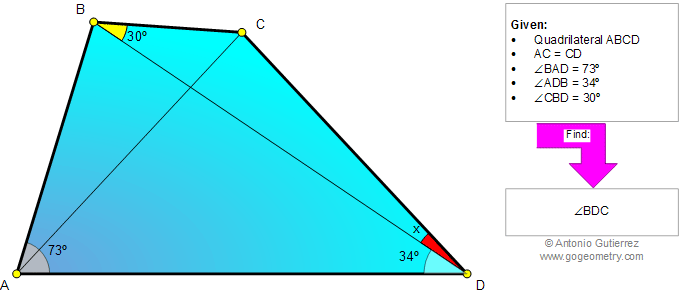Online Geometry Problem 830: Quadrilateral, Triangle, Angles, 30 degrees, Congruence. Level: High School, SAT Prep, College, Mathematics Education

 The figure below shows a quadrilateral ABCD with AC = CD, angle BAD = 73 degrees, angle ADB = 34 degrees, and angle CBD = 30 degrees. Find the measure of angle BDC. Try to use elementary geometry (Euclid's Elements).Home | Search | Geometry | Problems | All Problems | Open Problems | Visual Index | 10 Problems | 821-830 | Triangles | Quadrilaterals | Angles | 30 degrees | Email | Solution / comment | By Antonio Gutierrez